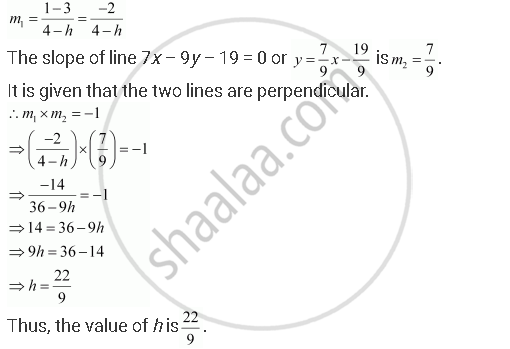# The Line Through the Points (H, 3) and (4, 1) Intersects the Line 7x – 9y – 19 = 0. at Right Angle. Find the Value of H. - Mathematics

The line through the points (h, 3) and (4, 1) intersects the line 7x – 9y – 19 = 0at right angle. Find the value of h.

#### Solution

The slope of the line passing through points (h, 3) and (4, 1) isConcept: General Equation of a Line
Is there an error in this question or solution?
Chapter 10: Straight Lines - Exercise 10.3 [Page 228]

#### APPEARS IN

NCERT Class 11 Mathematics
Chapter 10 Straight Lines
Exercise 10.3 | Q 10 | Page 228

Share